Next: Direct stop contribution at Up: fusion Previous: Direct stop contribution at

### Cycling contribution

For the cycling contribution, we take advantage of the fact that we had two independent Si detectors, and study the correlations between them. With an energy cut of 2501<E<3700ch and the time cut of 0.02<t<0.4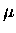s in one of the detectors, which selected alpha events with a high probability, we looked at the other detector.

With an energy cut in the second detector of 2701<E<3100 ch to select proton events, we obtained the coincidence rates with two different time cuts: (a)s, and (b)s, where is the time difference between the proton and the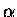candidate events. The yield with time cut (b) was corrected for the cut efficiency assuming the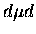fusion disappearance rate of 34s-1 given by the hyperfine transition rate in the kinetics model  , as well as the average time-of-flight difference between the 3 MeV proton and 3.5 MeV, while the cut (a) required no time corrections. The conditional solid angle for the protons given anis detected, could be different from the solid angle singles events, since thedetection biases the proton distribution towards the first detector ( i.e., away from the proton detector). This effect was estimated using a Monte Carlo  taking into account the geometrical correlation of the two detectors, and found to give about 10% (relative) reduction in the second detector. Using the coincidence solid angle, , theyield with the energy cut 2501<E<3700 ch, /GMU, and the time cut efficiency for cut (a) ( for the cut (b)), we obtain the proton yield per dt fusion from cycling, as

 (110)

where Ycoin is the coincidence yield per GMU, and we ignored the small sticking probability in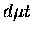fusion. The resulting from Target II-13 are given in Table 8.11.

Table: , the probability that the proton is produced after dt fusion is observed, is given for each coincidence pairs and the time cuts.
 Time cut Proton yield/dt fusion ( ) Si1(p) Si2() Si2(p) Si1() s 2.6 (4) 2.3 (4) s 0.9 (3) 0.9 (3)

The inconsistency between the results from the two time cuts can be due either to the time cut efficiency or possible presence of a prompt background (such asdecay electron). For example, if thefusion disappearance rate is different in a thin layer from 34s-1 measured in bulk solid targets, the time correction made is not appropriate. As well, with limited statistics, it is difficult to estimate the prompt background contribution. Given the uncertainty, we take the average of the two cuts, and quote an error covering two extremes of the error bars. Thus we have as the contribution from cycling. In our analysis below, we assume the cycling proton yield per dtto be independent of the D2 layer thickness, which is sufficient for the accuracy required here.

We note that at late time s, the coincidence yield is more than an order of magnitude smaller than at early times, hence the slow lifetime component of(observed in bulk solid and other targets) appears nearly absent from cycled fusion in a thin layer.

The value of here should be compared with our earlier estimate with the kinetics model given by (Eq. 8.3), which is significantly higher. It is interesting to note, however, that our value is more or less consistent with the estimated fast component yield in the kinetics model ( ). One possibility is that the muonic deuterium in the lower hyperfine state ( ) escapes from the layer before fusion takes place, since the low rate of non-resonant fusion ( s), which is responsible for the slow component, implies a rather long interaction length. Another possibility, of course, is that the muon escapes from the layer before stopping to form muonic deuterium . Physics of the muon cycling and the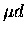transport in thin layers is a very interesting topic on its own, indeed.Next: Direct stop contribution at Up: fusion Previous: Direct stop contribution at